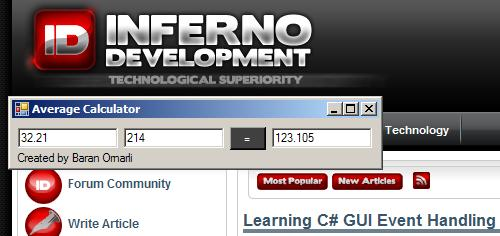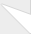# Creating C# Average Calculator

I had to calculate a lot of average numbers from various websites and so I decided to make a small average calculator that would calculate the average number of two input numbers in a fluid fashion. This average calculator works really well and is faster than using silly windows calculator or even excel.

toc_collapse=0;
Contents

Firstly, you need to open up Visual Studio C# or sharp develop. Create a new project and start a form design for windows applications.

### Designing the GUI

Next, design a calculator user interface (GUI) that fits your needs. Mine has 2 input boxes and one output box, but you can add more than that.Here's the code that visual studio came up with for my design! This is called Form1.Designer.cs (and in Visual Studio you only see it visually).

Don't forget to add Tab Index values to your input boxes for convenience!!! (start from 0!)

namespace AverageCalculator
{
partial class Form1
{
/// <summary>
/// Required designer variable.
/// </summary>
private System.ComponentModel.IContainer components = null;

/// <summary>
/// Clean up any resources being used.
/// </summary>
/// <param name="disposing">true if managed resources should be disposed; otherwise, false.</param>
protected override void Dispose(bool disposing)
{
if (disposing && (components != null))
{
components.Dispose();
}
base.Dispose(disposing);
}

#region Windows Form Designer generated code

/// <summary>
/// Required method for Designer support - do not modify
/// the contents of this method with the code editor.
/// </summary>
private void InitializeComponent()
{
this.button1 = new System.Windows.Forms.Button();
this.textBox1 = new System.Windows.Forms.TextBox();
this.textBox2 = new System.Windows.Forms.TextBox();
this.textBox3 = new System.Windows.Forms.TextBox();
this.SuspendLayout();
//
// button1
//
this.button1.BackColor = System.Drawing.SystemColors.ControlDarkDark;
this.button1.ForeColor = System.Drawing.SystemColors.ActiveCaptionText;
this.button1.Location = new System.Drawing.Point(218, 7);
this.button1.Name = "button1";
this.button1.Size = new System.Drawing.Size(36, 23);
this.button1.TabIndex = 2;
this.button1.Text = "=";
this.button1.UseVisualStyleBackColor = false;
this.button1.Click += new System.EventHandler(this.button1_Click);
//
// textBox1
//
this.textBox1.Location = new System.Drawing.Point(6, 9);
this.textBox1.Name = "textBox1";
this.textBox1.Size = new System.Drawing.Size(100, 20);
this.textBox1.TabIndex = 0;
//
// textBox2
//
this.textBox2.Location = new System.Drawing.Point(112, 8);
this.textBox2.Name = "textBox2";
this.textBox2.Size = new System.Drawing.Size(100, 20);
this.textBox2.TabIndex = 1;
//
// textBox3
//
this.textBox3.Location = new System.Drawing.Point(260, 8);
this.textBox3.Name = "textBox3";
this.textBox3.Size = new System.Drawing.Size(100, 20);
this.textBox3.TabIndex = 4;
//
// Form1
//
this.AutoScaleDimensions = new System.Drawing.SizeF(6F, 13F);
this.AutoScaleMode = System.Windows.Forms.AutoScaleMode.Font;
this.ClientSize = new System.Drawing.Size(367, 34);
this.Name = "Form1";
this.Text = "Average Calculator";
this.ResumeLayout(false);
this.PerformLayout();

}

#endregion

private System.Windows.Forms.Button button1;
private System.Windows.Forms.TextBox textBox1;
private System.Windows.Forms.TextBox textBox2;
private System.Windows.Forms.TextBox textBox3;
}
}

### Writing the Event Handling

Finally, we write our code that handles the GUI and events.

Because of partial classes, Visual Studio is able to split up The Form1 class into two different files (Form1.cs and Form1.Designer.cs)

using System;
using System.Collections.Generic;
using System.ComponentModel;
using System.Data;
using System.Drawing;
using System.Text;
using System.Windows.Forms;

namespace AverageCalculator
{
public partial class Form1 : Form
{
public Form1()
{
InitializeComponent();
}

private void button1_Click(object sender, EventArgs e)
{
double a = Convert.ToDouble(textBox1.Text.ToString());
double b = Convert.ToDouble(textBox2.Text.ToString());
textBox3.Text = Convert.ToString((a + b) / 2.0);
textBox1.Focus();
}
}
}

### Convert.ToDouble, double.Parse, Int32 Differences

Here's the important code, our event handling for the button1 (which is the equal sign):

private void button1_Click(object sender, EventArgs e)
{
double a = Convert.ToDouble(textBox1.Text.ToString());
double b = Convert.ToDouble(textBox2.Text.ToString());
textBox3.Text = Convert.ToString((a + b) / 2.0);
textBox1.Focus();
}

I declared the button name "button1" and so I use the event handling _Click to create the function.

I used double because I have a lot of decimal numbers to calculate. Int32 (int) could have been used but then decimal points would not work properly. If you use int, use Int32.Parse or Convert.ToInt32() to convert from string.

I use Convert.ToString to convert from double to string again.

If you get any errors like:
Cannot implicitly convert type 'double' to 'string'
Cannot implicitly convert type 'int' to 'string'
Cannot implicitly convert type 'string' to 'double'

It's because you did not properly convert your strings or doubles by using Convert or .Parse.

Yes, there is another way to do this, instead of
Convert.ToInt(), you can do Int32.Parse(), but the Int32 way will cause an exception if you give it a null argument (while the Convert method will just return zero). Just use the Convert method all the time.

There is also an Int32.TryParse() function that returns boolean and you can use an if statement to check for errors to avoid nasty Exceptions during your calculations.

if(Int32.TryParse(mystring)){
int a = Int32.Parse(mystring);
}

Maybe you can recreate this program in a more complex manner? Such as with 4-5 input boxes, or a button that automatically adds new input boxes for bigger averages.

Come by our forums and show us your code, we can help--you'll learn a lot.

### A more complex manner wouldA more complex manner would be having a multiline edit box and typing in each number on a separate line. Then, you could keep updating the average of all the numbers entered in another edit box or label. Good tutorial!

————

Get Linux or die tryin'

### Cool idea, maybe we could doCool idea, maybe we could do that and add it on to the end of the tutorial. Or maybe like an excel list box. Oh wow, I just thought of something cool!

You could have a table list that looks like excel, but let's say you enter 2 columns of numbers, then magically the third column should display average of the two.

Then if you add 2 rows of numbers (4x4 now), then two more numbers appear under column 1 row 3, and column 2 row 3, and those are averages of the above columns.

That way it would be faster than excel!

By the way, for our readers, please retweet for us, it would be greatly appreciated. If you have questions, feel free to leave comments.### Awesome article! This isAwesome article! This is really useful for accounting too in case you are frustrated with excel.Excellent never made a program that can actually be used in my daily life hehe.### What an easily understandableWhat an easily understandable tutorial, thank you. How would you modify this to do way more averages?### Well that was an interestingWell that was an interesting read, thank you for explaining.### C# is just so much fun. GOOOC# is just so much fun. GOOO C# .NET!### greatgreat### This one was the great postThis one was the great post man.It was really helpful for me.Thanks for the wonderful share.

Great
learn math### just return the computerjust return the computer average as double